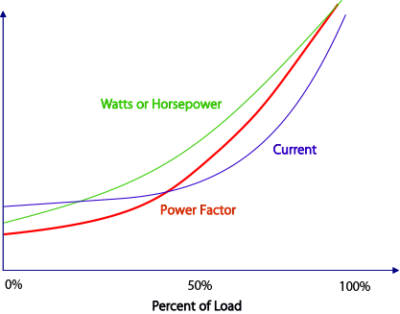High Performance Current Sensing for Automation Applications
1-800-959-4014 | sales@nktechnologies.com
Easily Monitor Wattage of Inductive LoadsResistive loads can be accurately measured by monitoring current only, but inductive loads such as motors and transformers may have current requirements that do not change as linearly as a strictly resistive load. Motors tend to have a low power factor when idling; the current draw changes very little from no load to roughly 50% of its rated load. The power factor improves as a motor load is increased to its design capacity, and as this occurs, the current reaches the motor’s Full Load Amperage rating (FLA). In many cases, the power factor of a motor improves 10 to 30% when comparing no load conditions to full load design conditions.

By measuring power rather than just current, the APT-TH power transducer produces an output proportional to watts consumed. There is a linear increase in output, as the wattage is a factor of voltage, current and power factor. For example, monitoring a 200 horsepower, 480 volt 1800 RPM motor. The nameplate shows FLA of 233 amps and power factor of 85%. No load (open shaft or magnetizing current) in 4-pole squirrel cage induction motors runs about 25% of the FLA. Power factor at no load can be as low as 0.20. At about one-half load, the current used begins to rise as the load increases. For illustrative purposes, this motor draws 233 X 0.25 amps with no load (58.25 amps), and the power factor might be 0.20. At 480 volts, this is roughly 9,685 watts. At half load, assuming little increase in current, the power factor would be closer to 0.75, or 36,320 watts. At full load it is 164,651 watts (233 X 0.85 x 480 X1.732). While neither the current nor the power factor are linear changes, the watts consumed increase in a much more linear manner.The APT-TH can be used to monitor any AC loads. However, in conditions where the torque requirements change as work is fed into a machine, such as machine tools, lathes and drill presses, the horsepower (wattage) requirement is reflected in the transducer’s output. As the tool hits the work surface, the load increases. This is shown as an increase in watts, whereas it might not be shown as an increase in current as significantly. Monitoring wattage is also a better way to analyze a system with oversized motors, such as conveyor belts where product is loaded onto the belt in varying quantities. The design torque must be much higher to overcome temporary overloads, so the power factor will often be low while the belt is lightly loaded.

###APT-TH Series Power Transducers for Monitoring Inductive Load Wattage

APT-TH Power Transducers provide a simple, accurate and compact means of monitoring both the current and the voltage of a load. By comparing the current waveform with the voltage waveform, a true representation of the actual wattage (horsepower) being consumed can be provided by the APT-TH transducer in the form of a 4–20 mA or 0–5VDC signal.

### Related Products

Registration Required